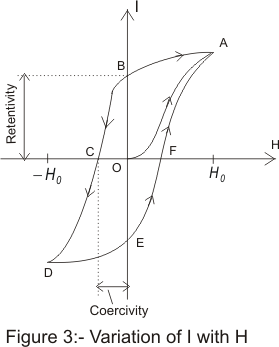# hysteresis

## Hysteresis

• We have already mentioned that in case of ferromagnetic materials ,the relation between I and H is not linear
• This relation can even depend on the history of the sample i.e whether it has been previously magnetized or not
• when we place a ferromagnetic material in the magnetic field it gets magnetized by induction
• If the field strength is first increased from a zero to high value and then decreased again, it is observed that the original curve is not retraced, the induction lags behind and follows a characteristics curve. This phenomenon is known as Hysteresis and characteristics curve is known as hysteresis loop as shown below in the curveFigure 3 shows the variation of I and H.In the beginning I= 0 and H=0 as represented by the point O in the figure. At this instant the sample is in unmagnetized state
• As value of H is increased, I also increases non uniformly .If we increase H indefinitely the intensity of magnetization of ferromagnetic material approaches finite limit known as saturation
• Thus at H=H0 ,the magnetization becomes nearly saturated and magnetization and magnetization varies along path OA
• Now if we begin to decrease the value of magnetic field ,the magnetization I of the substance also begin to decrease but this time not following the path AO but following a new path AB
• when H becomes equal to zero, I still have value equal to OB,This magnetization remaining in substance when magnetizing field becomes equal to zero is called the residual magnetism and the remaining value of I at point B is known as retentively of the material
• To reduce I to zero, we will increase field H in reverse direction and the magnetization I decreases following curve BC where at point C,I becomes equal to zero where H=OC
• The value OC of the magnetizing field is called coercivity of the substance
• the coercivity of the substance is a measure of the reverse magnetizing field required to bring magnetization I equal to zero
• if we further increase H beyond OC the sample begins to get magnetized in reverse direction ,again getting saturated at D at H=-H0
• while taking back H from its negative value through zero to its original maximum positive value H0,we symmetrical curve DEFA
• Thus we see that if the field strength is first increased from zero to saturated and then decreased again, it is observed that original curve is not retraced, the induction lags behind the field and follows a characteristic curve .This phenomenon is known as hysteresis and the characteristic curve ( Here ABCDEFA) is known as Hysteresis loop